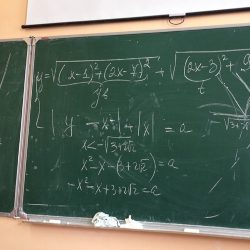# Creating Algebraic Expressions: Algebra - Grade 9Subject Pre-Algebra or Algebra 1

Lesson Objective: Students will discover how to create variables and use them in mathematical expressions. This is a game to get students to begin thinking about building algebraic expressions and equations before introducing variables.

Common Core Standard

CCSS.MATH.CONTENT.HSA.CED.A.1: Create equations and inequalities in one variable and use them to solve problems. Include equations arising from linear and quadratic functions, and simple rational and exponential functions.

## Materials

• Different types of calculators including those on a computer
• Optional materials to enhance gameplay.
• As you play, you may find that adding silliness to the game will make it more successful. Give someone a microphone (real or pretend, it does not matter) to hold in front of a speaker. Use applause, either from the audience or pre-recorded. Assign a student to start and stop the applause button. Have students record the contest and put it on YouTube. Allow trash talk. Give teams names such as Tadpoles vs. Toads and the contest is the Swamp Bowl.

## Starter:

Begin by setting up your teams. There are lots of variations to the game setup. Two teams, teacher vs. students, individual contestants, etc. Assign one student (or one team) the task of choosing a number and writing it down. He is called Mr. Chooser, Madam Chooser, or some creative title.

The "Builder" builds a sequence of instructions such as "add three," "double it," etc. Then the Chooser tells their results and the audience (second team, two contestants, etc.) has to determine the original number.

Early on, the instructor should be the Builder as she can determine the difficulty. Start with simple, one-step tasks, probably addition or subtraction. Gradually build to multiplication/division, fractions, etc. Then on to two or three-step sequences. If students are allowed to build the sequence, they may randomly give steps such as divide by three, resulting in fractional answers. Prepare for this.

For Example:

Instructor (dramatically): "Mr. Chooser, are you ready?"

Chooser: "Yes, I am."

Instructor: "Choose a number less than 20 and write it down. Do you have your number?"

Chooser: "Yes, I do."

Instructor: "Now add nine and tell me the results."

Chooser: "23"

Instructor: "Team 2?"

Instructor: "Yes, that is correct! Score Keeper, add one for Team 2."

## Main

Let students in Team 2 (the responding team) work together, but have a designated Responder. At first, the answer to the easy sequence will be obvious and the Responder will call it out. When more difficult, they may wait to find a consensus. When there is not agreement, they will review to see what is right.

Gradually increase the difficulty of the instruction sequence so that Team 2 students cannot easily do the math in their heads and will need to write the steps down. This, of course, is the heart of math, recording abstract concepts in symbolic notation. It is likely that at first students will write simple words for the math verbs such as "add" as opposed to the + symbol, and "times" for multiply, but the words subtract and divide cannot be written quickly. They may develop shorthand but more likely will use symbols.

If students have already experienced variables, they will likely begin using them. If not, they may develop their own notation, using such symbols as a box □, open/close parentheses (), or more likely the letter N for the Chooser's unknown number.

Once you determine the variables they are using, make the notation public (write it on the whiteboard). You can allow an extra point if the expression is correct. If students have invented their own notation such as □ + 3, stick with it for the time being.

Eventually, introduce sequences that allow discussions of inclusion in notation. For example, "Start with a number. Add 3. Double it." Students will likely write, "N + 3 x 2" and will use the strategy of doing each step in the sequence backward. If Chooser starts with eight and then states that his result is 22, Team 2 will divide by 2 and then subtract 3, which will give 8, the right answer.

However, if the Chooser uses a scientific calculator and types "8 + 3 x 2" he will get 14, the correct answer if the calculator uses algebraic Logic. (You may want to intentionally provide the Chooser with an Algebraic-Logic calculator at the beginning to anticipate this dilemma.) So Chooser declares his answer is 14 but Team 2 students using the reverse-operation process believe his original number should be 4 (14 / 2 – 3).

Don't be in a hurry to resolve the contradiction. Let the students find which calculators/computers give which answer and look for commonalities. Eventually, you hope they ask you (the expert) which is correct.

Your answer should be, do you want to do the addition or multiplication first? Their answer should be to do whatever comes first (from left to right).

So then you can ask, how would you show if you wanted to do the multiplication first, i.e., "8 added to the product of 3 times 2." It is likely someone will suggest using parentheses. 8 + (3 x 2).

Gradually begin calling Chooser's "unknown number" the "variable number" and then just the "variable."

This game has a vast amount of opportunities for discovery moments. A student may observe that it does not matter if the add 8 comes after the (3 x 2).

Optional (either in the same class period, but most likely in a subsequent class)

Gradually begin using x as the symbol of your unknown. This will seem logical to most students as x is often the representation of something unknown.

Notation that uses x as both an unknown and the symbol for multiplication will be confusing. For example, go back to "Start with a number. Add 3. Double it." x + 3 x 2 is quite confusing.

This should lead to the notation of showing multiplication before an opening parenthesis. ie 2(x + 3).

Written by James Zimmer

Education World Contributor